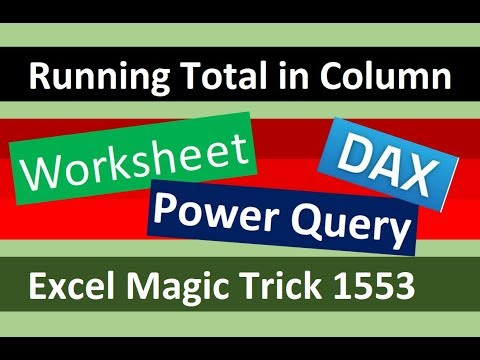Select Page

Entire page with all Excel Files for All Videos: http://people.highline.edu/mgirvin/excelisfun.htm

In this video learn how to create a Running Total (Cumulative Totals) in a Column using Excel Worksheet Formulas, Power Query M Code or a DAX Calculated Column in Power BI or Excel Power Pivot Data Model. Learn how to create Overall Running Total and Running Totals by Month. Learn the important skill of creating Expandable Ranges in a Worksheet Formula, DAX Formulas and Power Query Formulas.
Topics:
1. (00:07) Introduction
2. (01:20) Excel Worksheet Formula solution using SUMIFS and EOMONTH functions with an Expandable Range. Two formulas: Overall Running Total and Running Totals by Month.
3. (04:32) Look at expandable range in a Worksheet Formula
4. (05:18) Import Table for both Power Query Solution and DAX Solution using Power Query and the Reference feature.
5. (06:50) Power Query Custom Column with Table.AddColumn Function, Table.SelectRows Function and Two M Code Custom Functions to create expandable ranges that can calculate a Overall Running Total and Running Totals by Month in a Column.
6. (08:15) Lesson about how Custom Columns and M Code Custom Functions work, including the how the keyword “each” is a substitute for an explicitly defined Custom Function.
7. (13:33) Look at expandable range in a Power Query Formula.
8. (14:25) Field Access Operator for Looking up: 1) A Full Column or 2) A Single Item from Each Row.
9. (20:01) DAX Calculated Column with the functions CALCULATE, SUM, FILTER, EARLIER, EOMONTH and a Var Variable to create expandable ranges that can calculate a Overall Running Total and Running Totals by Month in a Column.
10. (26:46) How to use a Variable in DAX. See Var = Mon Return syntax for a Variable in DAX.
11. (30:26) SummaryRead More